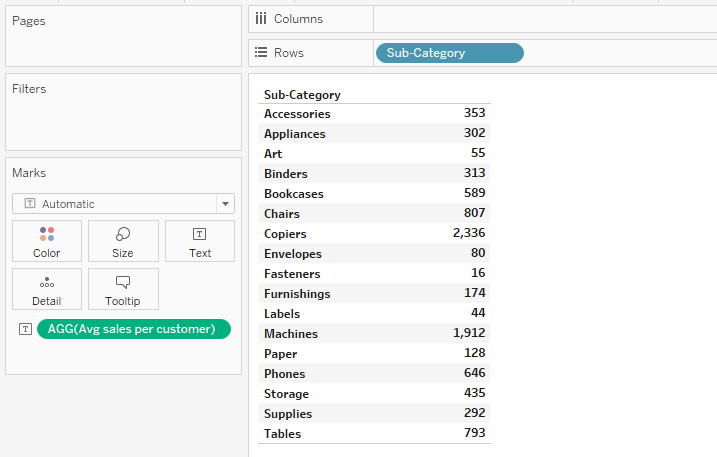top of page
Search
• Bernard K

# How to use INCLUDE level of detail (LOD) expression in Tableau

Updated: Mar 4In the previous article – I demonstrated to you how to use EXCUDE level of detail expression in Tableau. You can visit that article on EXCLUDE LOD using link below.

In this article, we’re going to explore the INCLUDE level of detail expression. INCLUDE level of detail expression computes values using the specified dimensions - in addition to other dimensions present in the view. (Meaning the dimensions used to compute the INCLUDE level of detail expression will change when you add or remove fields from the view).

See in the example below - which demonstrates a use case of INCLUDE level of detail expressions.

### Example: Computing average sales per customer

Let’s say using Sample-Superstore data set, you would like to compute the average sales per customer for different product Sub-categories.

One solution to this problem is.

• Drag Sub-Category to the rows.

• Create an arithmetic calculation by summing all sales divided by all customers. As shown below.Drag the above calculation to the label shelf.

(Which gives us the average sales per customer for each product sub-category)The same problem could also be solved using the INCLUDE level of detail expression as shown below.

This is how the formula will look like.When dragged to the view, this expression computes the sales on per Customer_ID basis and then aggregates the values at Sub-Category level.

And when we aggregate this expression using average – we’ll basically be computing the average sales per customer for each sub-category.

Let’s test that by dragging the above calculation to the view – and change the aggregate to average.From the resulting view – you can see that the two expressions give us the same results. (That is, both are computing the average sales per customer for each sub-category).

If you like the work we do and would like to work with us, drop us an email on our Contacts page and we’ll reach out!